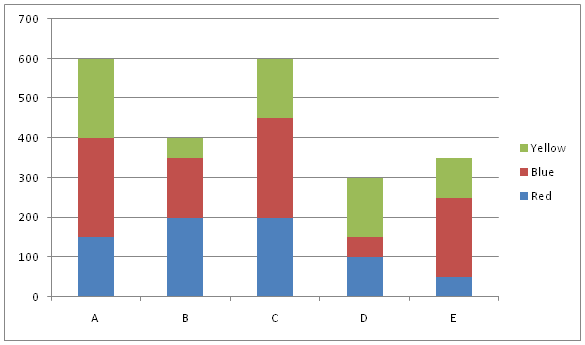# SBI PO Prelims Quantitative Aptitude (Day-14)

Dear Aspirants, Our IBPS Guide team is providing new series of Quantitative Aptitude Questions for SBI PO 2020 so the aspirants can practice it on a daily basis. These questions are framed by our skilled experts after understanding your needs thoroughly. Aspirants can practice these new series questions daily to familiarize with the exact exam pattern and make your preparation effective.

Start Quiz

Ensure Your Ability Before the ExamTake SBI PO 2020 Prelims Free Mock            Test

Direction (01 – 05): Study the following information carefully and answer the given questions:

The given bar graph shows the number of employees like three different colors-Red, Blue and Yellow from five different companies-A, B, C, D and E.1) The sum of the number of employees like Red color from company A and Blue color from company C together is approximately what percent of the sum of the number of employees like Yellow from company D and Red color from company B together?

A) 110%

B) 114%

C) 118%

D) 120%

E) None of these

2) If out of the total employees, 25%, 50% and 40% of employees are female who like Yellow from company E, D and C respectively, then what is the total number of males from these three companies together?

A) 240

B) 280

C) 320

D) 360

E) None of these

3) Find the average number of employees like Blue in all the companies together?

A) 160

B) 170

C) 180

D) 190

E) None of these

4) If the number of employees like Yellow in company B is increased by 50% and the number of employees like Red in company C is increased by 10%, then what is the difference between the total number of employees in company B and C?

A) 145

B) 155

C) 175

D) 195

E) None of these

5) What is the difference between the number of employees who like Red in all the companies together and the number of employees who like Yellow in all the companies together?

A) 30

B) 40

C) 50

D) 60

E) None of these

6) If the shopkeeper was earned a profit of 20% by selling the mobile after the discount of 25% and the marked price of the mobile isRs.8000, then find the cost price of the mobile.

A) Rs.5000

B) Rs.6000

C) Rs.4000

D) Rs.7000

E) None of these

7) The boat travelled 36 km downstream and 12km upstream in 5 hours and also the boat travelled 18 km downstream and 12km upstream in 4 hours. What is the speed of stream?

A) 7 kmph

B) 7.65kmph

C) 6.25kmph

D) 9.25kmph

E) None of these

8) If the certain sum is invested in scheme A which is offering simple interest at 18% per annum for 5 years and the same amount is invested in scheme B which is offering compound interest at 10% per annum for 2 years, then find the ratio of simple interest to compound interest on the sum.

A) 23:9

B) 27:7

C) 28:9

D) 30:7

E) Cannot be determined

9) The box contains 3 red, 2 green, 4 yellow and 6 blue balls. If four balls are picked at random, what is the probability of one of them is green, 2 is blue and one is red?

A) 7/81

B) 5/91

C) 6/91

D) 8/91

E) None of these

10) Four years ago, the average ages of 5 persons A, B, C, D and E is a prime number. If A’s age is 50% of the age of C and after 12 years the ratio of the ages of B to D is 3:2, then find the present age of E.

A) 30 years

B) 20 years

C) 18 years

D) 24 years

E) Cannot be determined

Directions (1-5) :

Required percentage= [(150+250)/(150+200)]*100

=114%

Male in company E=75/100*100=75

Male in company D=50/100*150=75

Male in company C=150*60/100=90

Required Total=75+75+90=240

Required average= (250+150+250+50+200)/5

=180

Yellow in company B=50*150/100=75

Red in company C=200*110/100=220

Required Difference=(220+250+150)-(200+150+75)

=195

Required Difference=(150+200+200+100+50)-(200+50+150+150+100)

=50

Directions (6-10) :

CP=8000*75/100*100/120

= Rs. 5000

Let Speed of downstream=x

And Speed of upstream=y

36/x+12/y=5———-(1)

18/x+12/y=4————(2)

After solving this we have

x=18kmph and y=4 kmph

Speed of stream=(18-4)/2=7 kmph

Required ratio=(x*5*18/100):(x*(1+10/100)2-x)

=90x/100:21x/100

=30:7

Required probability=2C1*6C2*3C1/15C4

=6/91